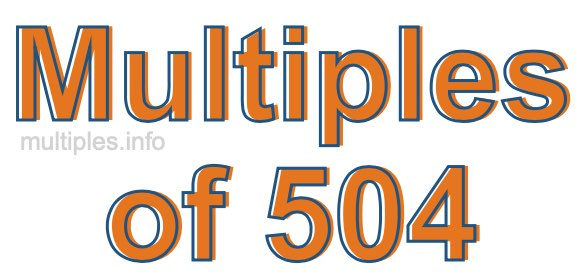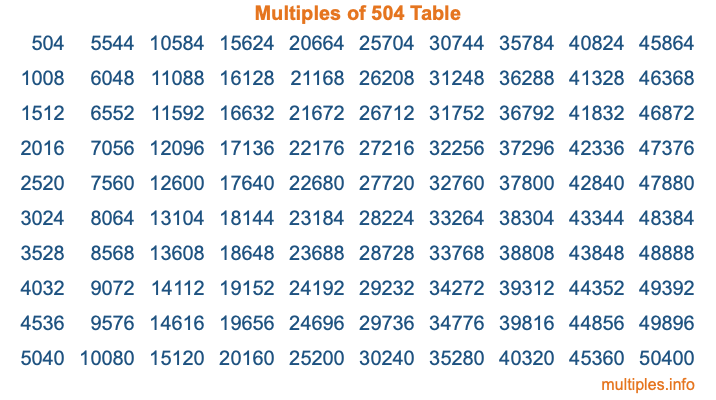Multiples of 504Welcome to the Multiples of 504 page. Here we will first teach you everything you will ever need to know about the multiples of 504, and then give you a study guide summary of everything we taught you to make sure you remember it all. Use this page to look up facts and learn information about the multiples of 504. This page will make you a multiples of five hundred four expert!

Definition of Multiples of 504
Multiples of 504 are all the numbers that when divided by 504 equal an integer. Each of the multiples of 504 are called a multiple. A multiple of 504 is created by multiplying 504 by an integer.

Therefore, to create a list of multiples of 504, you start with 1 multiplied by 504, then 2 multiplied by 504, then 3 multiplied by 504, and so on for as long as you want. Thus, the list of the first five multiples of 504 is 504, 1008, 1512, 2016, and 2520. To see a larger list of multiples of 504, see the printable image of Multiples of 504 further down on this page. We also have a category where you can choose any nth multiple of 504.

Multiples of 504 Checker
The Multiples of 504 Checker below checks to see if any number of your choice is a multiple of 504. In other words, it checks to see if there is any number (integer) that when multiplied by 504 will equal your number. To do that, we divide your number by 504. If the the quotient is an integer, then your number is a multiple of 504.

Is  a multiple of 504?

Least Common Multiple of 504 and ...
A Least Common Multiple (LCM) is the lowest multiple that two or more numbers have in common. This is also called the smallest common multiple or lowest common multiple and is useful to know when you are adding our subtracting fractions. Enter one or more numbers below (504 is already entered) to find the LCM.

Check out our LCM Calculator if you need more details about the Least Common Multiple or if you need the LCM for different numbers for adding and subtraction fractions.

nth Multiple of 504
As we stated above, 504 is the first multiple of 504, 1008 is the second multiple of 504, 1512 is the third multiple of 504, and so on. Enter a number below to find the nth multiple of 504.

th multiple of 504

Multiples of 504 vs Factors of 504
504 is a multiple of 504 and a factor of 504, but that is where the similarities end. All postive multiples of 504 are 504 or greater than 504. All positive factors of 504 are 504 or less than 504.

Below is the beginning list of multiples of 504 and the factors of 504 so you can compare:

Multiples of 504: 504, 1008, 1512, 2016, 2520, etc.

Factors of 504: 1, 2, 3, 4, 6, 7, 8, 9, 12, 14, 18, 21, 24, 28, 36, 42, 56, 63, 72, 84, 126, 168, 252, 504

As you can see, the multiples of 504 are all the numbers that you can divide by 504 to get a whole number. The factors of 504, on the other hand, are all the whole numbers that you can multiply by another whole number to get 504.

It's also interesting to note that if a number (x) is a factor of 504, then 504 will also be a multiple of that number (x).

Multiples of 504 vs Divisors of 504
The divisors of 504 are all the integers that 504 can be divided by evenly. Below is a list of the divisors of 504.

Divisors of 504: 1, 2, 3, 4, 6, 7, 8, 9, 12, 14, 18, 21, 24, 28, 36, 42, 56, 63, 72, 84, 126, 168, 252, 504

The interesting thing to note here is that if you take any multiple of 504 and divide it by a divisor of 504, you will see that the quotient is an integer.

Multiples of 504 Table
Below is an image of the first 100 multiples of 504 in a table. The table is in chronological order, column by column. The first column has the first ten multiples of 504, the second column has the next ten multiples of 504, and so on.The Multiples of 504 Table is also referred to as the 504 Times Table or Times Table of 504. You are welcome to print out our table for your studies.

Negative Multiples of 504
Although not often discussed or needed in math, it is worth mentioning that you can make a list of negative multiples of 504 by multiplying 504 by -1, then by -2, then by -3, and so on, to get the following list of negative multiples of 504:

-504, -1008, -1512, -2016, -2520, etc.

Multiples of 504 Summary
Below is a summary of important Multiples of 504 facts that we have discussed on this page. To retain the knowledge on this page, we recommend that you read through the summary and explain to yourself or a study partner why they hold true.

There are an infinite number of multiples of 504.

A multiple of 504 divided by 504 will equal a whole number.

504 divided by a factor of 504 equals a divisor of 504.

The nth multiple of 504 is n times 504.

The largest factor of 504 is equal to the first positive multiple of 504.

504 is a multiple of every factor of 504.

504 is a multiple of 504.

A multiple of 504 divided by a divisor of 504 equals an integer.

504 divided by a divisor of 504 equals a factor of 504.

Any integer times 504 will equal a multiple of 504.

Multiples of a Number
Here you can get the multiples of another number, all with the same attention to detail as we did for multiples of 504 on this page.

Multiples of
Multiples of 505
Did you find our page about multiples of five hundred four educational? Do you want more knowledge? Check out the multiples of the next number on our list!